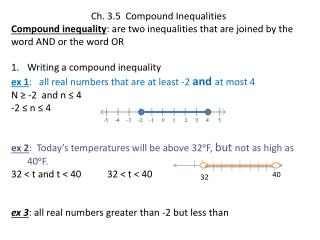DownloadDownload PresentationCh. 3.5 Compound Inequalities

# Ch. 3.5 Compound Inequalities

Download Presentation## Ch. 3.5 Compound Inequalities

- - - - - - - - - - - - - - - - - - - - - - - - - - - E N D - - - - - - - - - - - - - - - - - - - - - - - - - - -
##### Presentation Transcript

1. Ch. 3.5 Compound Inequalities Compound inequality: are two inequalities that are joined by the word AND or the word OR Writing a compound inequality ex 1: all real numbers that are at least -2 and at most 4 N ≥ -2 and n ≤ 4 -2 ≤ n ≤ 4 ex 2: Today’s temperatures will be above 32ᵒF, but not as high as 40ᵒF. 32 < t and t < 40 32 < t < 40 ex 3: all real numbers greater than -2 but less than 40 32

2. Practice All real numbers that are at least 2 and at most 9 All real numbers that are between -3 and 8

3. Solving a compound inequality containing AND ex: Solve -4 < r – 5 ≤ -1. Graph your solution Write the compound inequality as two inequalities joined by and. -4 < r – 5 and r – 5 ≤ -1 +5 +5 + 5 + 5 1 < r and r ≤ 4

4. Solving a Compound Inequality Containing AND Ex 2: Solve -6 ≤ 3x < 15 Write my two inequalities-6 ≤ 3x and 3x < 15 3 3 3 3 -2 ≤ x and x < 3 -2 ≤ x < 3

5. Practice -3 < 2x – 1 < 7

6. Practice 7 < -3n + 1 ≤ 13

7. Solving inequalities in word problems The acidity of the water in a swimming pool is considered normal if the average of three pH readings is between 7.2 and 7.8, all together. The first two readings for a swimming pool are 7.4 and 7.9. What are possible values for the third reading p will make the average pH normal? How do I find average? Where does my average have to beinbetween? 7.2 ≤ ( 7.4 + 7.9 + p) ≤ 7.8 3 21.6 ≤ 7.4 + 7.9 + p ≤ 23.4 21.6 ≤ 15.3 + p ≤ 23.4 (3) (3) (3) I first need to get 3 out of the denominator in order to get p all by itself!

8. 21.6 ≤ 15.3 + p ≤ 23.4 Break into two inequalities 21. 6 8 ≤ 15.3 + p and 15.3 + p ≤ 23.4 - 15.3 - 15.3 - 15.3 -15.3 6.3 ≤ p and p ≤ 8.1

9. Practice Suppose the first two readings for the acidity of the water in a swimming pool are 7.0 and 7.9. What possible values for the third reading will make the average pH normal?

10. Homework Pages 163-164 #’s 1-19 POW DUE TOMORROW!!!!!!!!!!